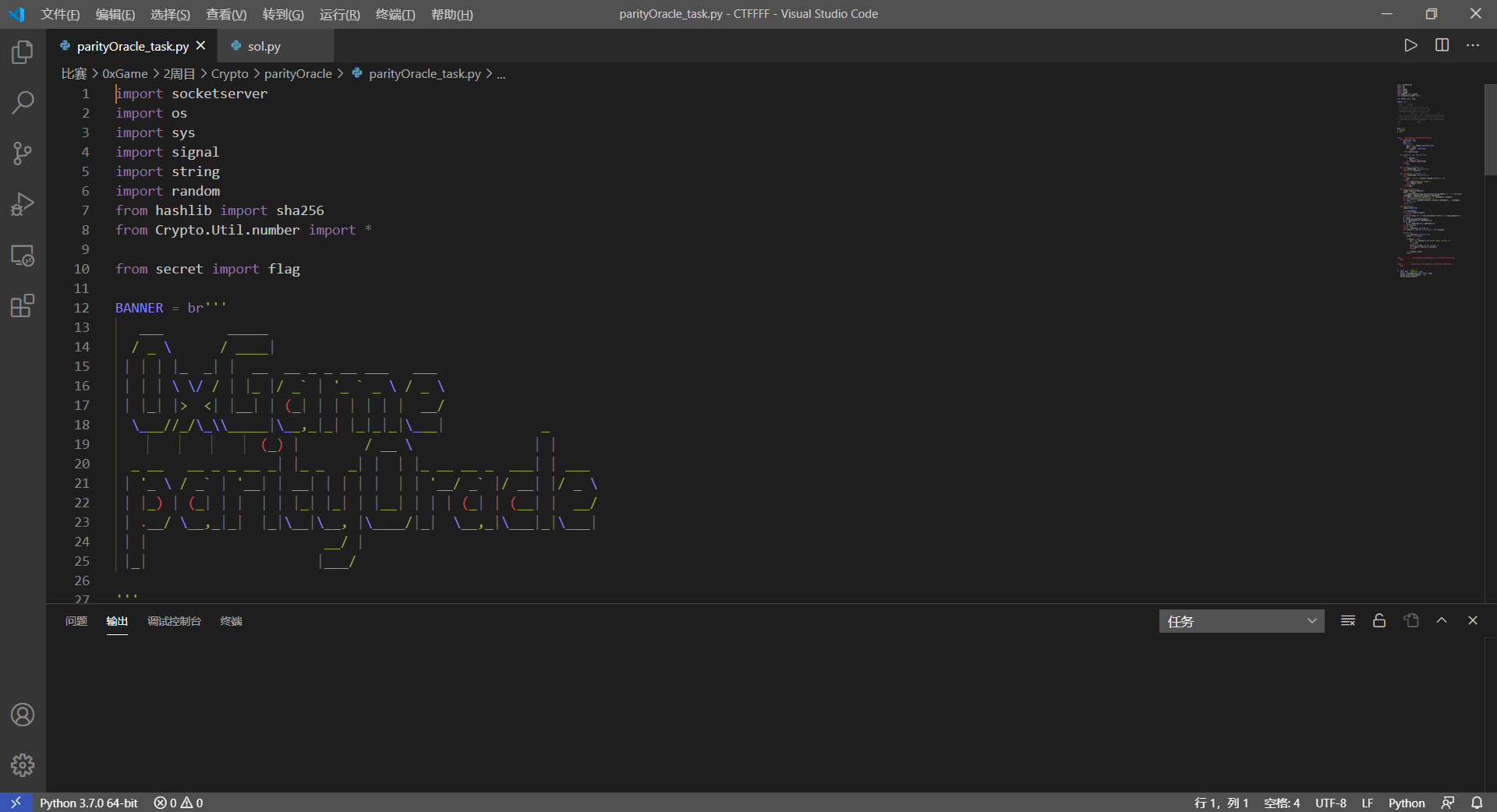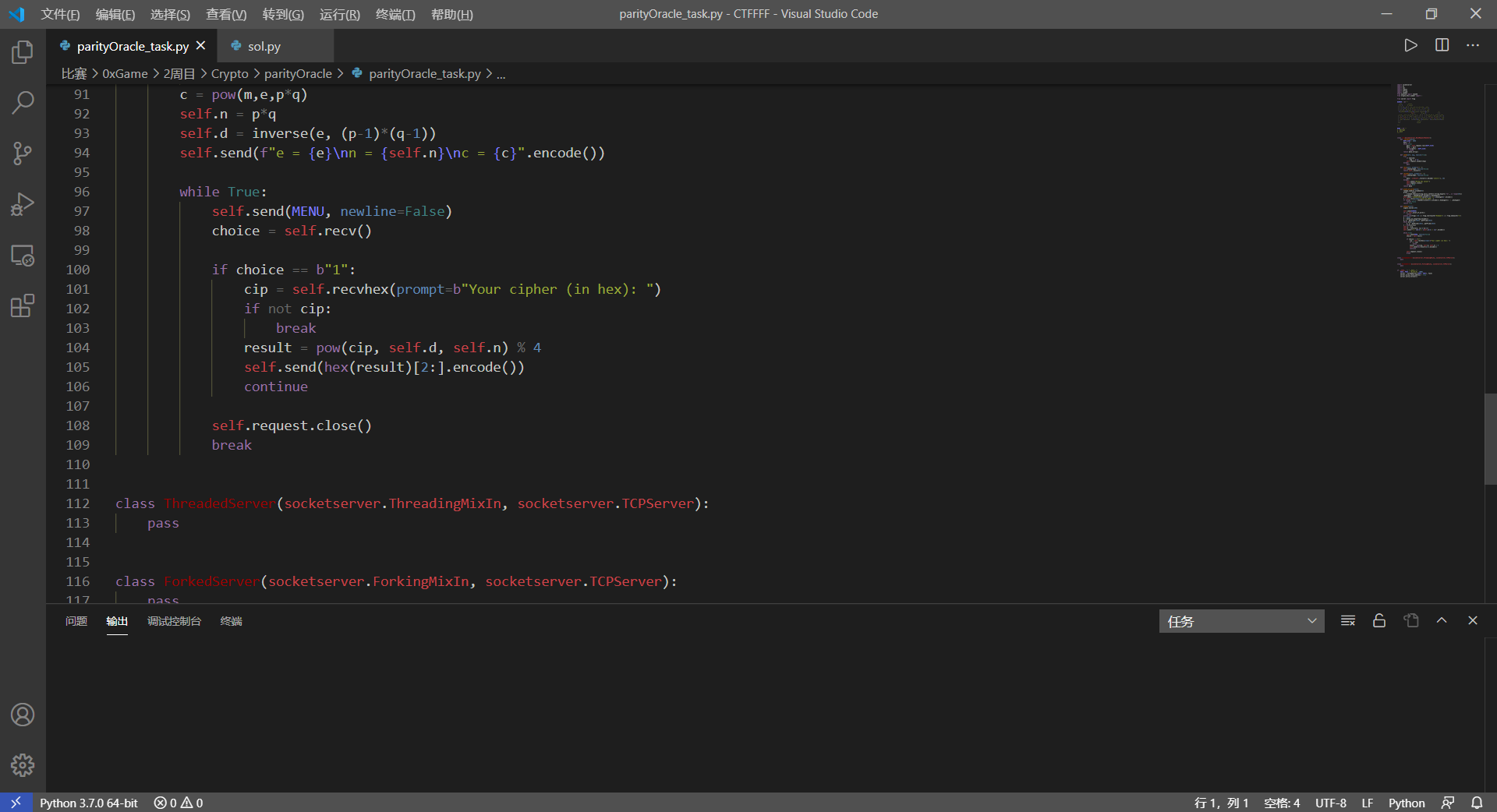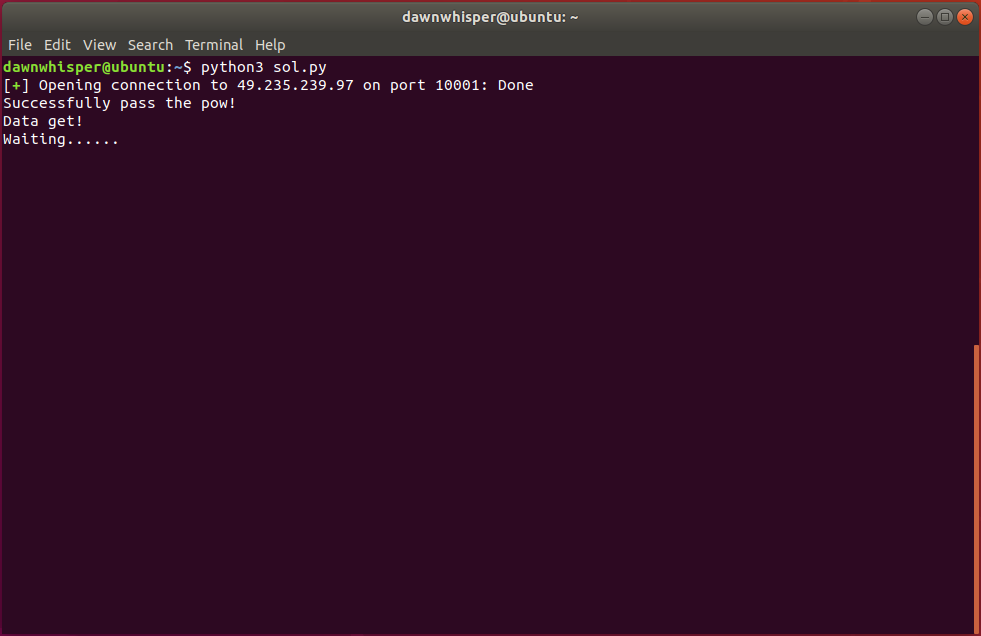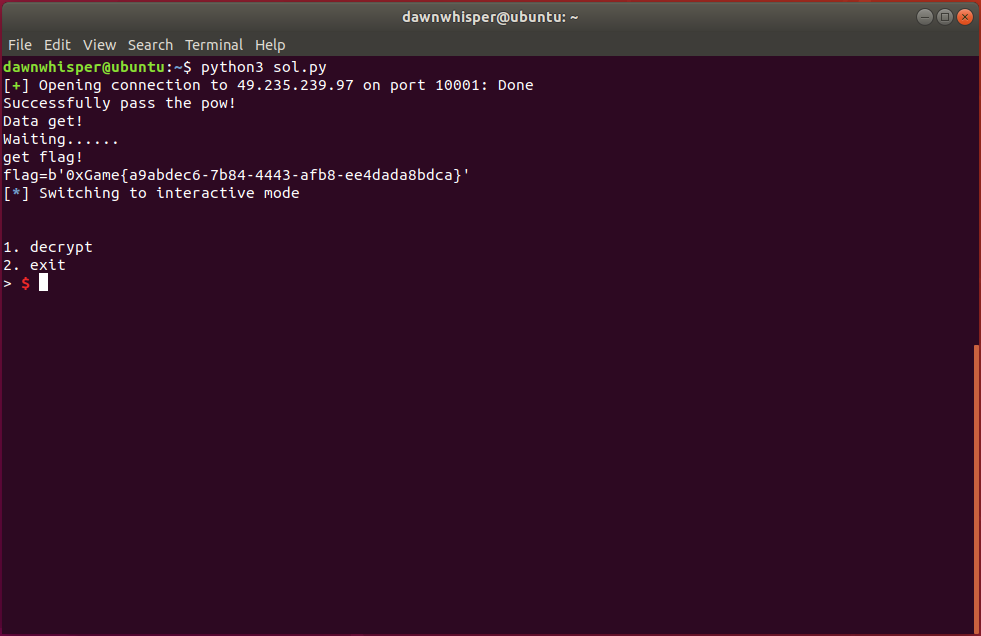RSA Parity Oracle

Dawn_whisper和他的辣鸡代码成长史（雾

原理

第一步

• 如果返回0，说明$2m < n$，因为乘2之后必定是一个偶数，膜2之后一定等于0（亦可以理解为2m变为二进制后最低位是0），也就说明$0 < m < \dfrac{n}{2}$
• 如果返回1，说明$2m > n$，因为乘2之后必定是一个偶数，而n一定是一个奇数（俩质数相乘肯定是个奇数），返回1的原因是$2m$在膜$n$时，因为比n大，所以会变成$2m-n$，这样得到的就是一个奇数，膜2之后一定等于1（2m变为二进制后最低位是1），也就说明$\dfrac{n}{2} < m < n$

第二步

• 我们假定$\dfrac{3\cdot n}{4}< m <n$，而通过第一次我们的分析我们得知$\dfrac{n}{2} < m < n$，接下来该怎么办呢？

• 如果假定$\dfrac{n}{2}< m<\dfrac{3\cdot n}{4}$，我们就会得到0！

……

例题

1.2020 0xGame Crypto parityOracle• 如果返回0，则$0< m<\dfrac{n}{4}$
• 如果返回1，则$\dfrac{n}{4}< m<\dfrac{n}{2}$
• 如果返回2，则$\dfrac{n}{2}< m<\dfrac{3\cdot n}{4}$
• 如果返回3，则$\dfrac{3\cdot n}{4}< m<n$

sol.py

running……